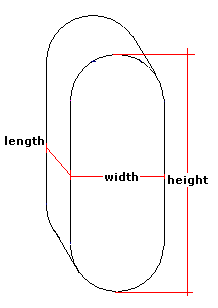Spike's Calculators

# Horizontal Oval On-Edge Tank Volume and Surface Area - CentimetresCalculate the volume and capacity of an on-edge horizontal oval tank or container. The oval tank used here has a rectangular shape with two semi-circular ends. This calculator uses centimetres for measurements.

##### Approximate weight of Fuel or Water
```one litre of water = 1 kilogram
one litre of diesel fuel = 0.832 kilogram
one litre of gas = 0.75 kilogram
```
##### Note:
`The width measurement has to be smaller than the height measurement!`

### Oval Tank Horizontal - On-Edge - Centimetres

Height of the Tank cm
Tank Width cm
Length of the Tank cm
Liquid Weight-Kilograms per Litre kg/L

#### Results:

###### Tank Volume
In Cubic Centimetres cm³
In Cubic Metres
In Cubic Inches in³
In Cubic Feet ft³
###### Tank Capacity
In Centilitres cl
In Litres L
In US Gallons gal US
In Imperial Gallons gal Imperial
###### Liquid Weight and Surface Area
Weight of Liquid kg
Surface Area in Square Centimetres cm²
Surface Area in Square Metres
Surface Area in Square Inches in²
Surface Area in Square Feet ft²

#### Calculator

1. enter the height of the on-edge horizontal oval tank in centimetres
2. the width of the tank in centimetres
3. the length of the tank in centimetres
4. the weight of the liquid in kilograms per litre

#### Results

1. the volume of the tank in cubic centimetres
2. the volume in cubic metres
3. the volume in cubic inches
4. the volume in cubic feet
5. the capacity of the tank in centilitres
6. the capacity in litres
7. the capacity in US gallons
8. the capacity in Imperial gallons
9. full tank liquid weight based on kilograms/L
10. the surface area of the tank in square centimetres
11. the surface area in square metres
12. the surface area in square inches
13. the surface area in square feet

##### Formula
```V  = (π R² + 2RS)L
where V is the volume
Π = 3.14159265
R half the width of the tank
S the height minus the width
L the length
```
##### Conversions
```one cubic centimetre (cm³) = 0.1 centilitres (cl)
one cubic centimetre (cm³) = 0.001 litres (L)
one cubic centimetre (cm³) = 0.000001 cubic metres (m³)
one cubic centimetre (cm³) = 0.000264172052358148 gallons (gal) US
one cubic centimetre (cm³) = 0.0610237440947323 cubic inches (in³)
one cubic centimetre (cm³) = 0.0000353146667214886 cubic feet (ft³)
one cubic centimetre (cm³) = 0.000264172052358148 gallons (gal) US
one cubic centimetre (cm³) = 0.000219969248299088 gallons (gal) Imperial
one square centimetre (cm²) = 0.0001 square metres (m²)
one square centimetre (cm²) = 0.15500031000062 square inches (in²)
one square centimetre (cm²) = 0.00107639104167097 square feet (ft²)
```

### Oval-Horizontal Tank (On-Edge)#### Filled